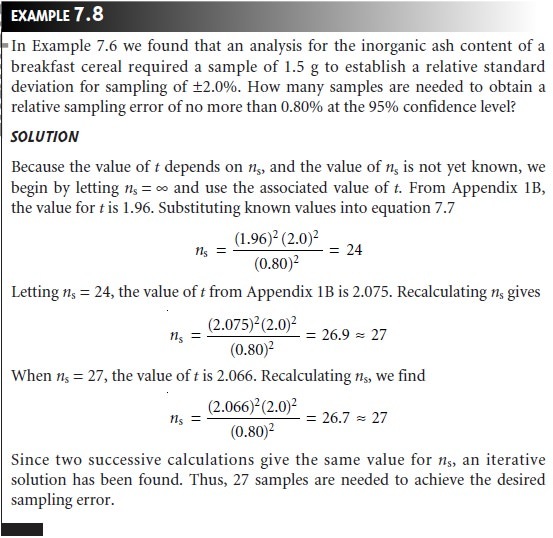Home | | Modern Analytical Chemistry | How Many Samples to Collect

# How Many Samples to Collect

In the previous section we considered the amount of sample needed to minimize the sampling variance.

How Many Samples to Collect

In the previous section we considered the amount of sample needed to minimize the sampling variance. Another important consideration is the number of samples required to achieve a desired maximum sampling error. If samples drawn from the target population are normally distributed, then the following equation describes the confidence interval for the sampling errorwhere ns is the number of samples and ss is the sampling standard deviation. Rear- ranging and substituting e for the quantity (ÎĽâ€“ Xâ€“), gives the number of samples as7.7

where ss2 and e2 are both expressed as absolute uncertainties or as relative uncertain- ties. Finding a solution to equation 7.7 is complicated by the fact that the value of t depends on ns. As shown in Example 7.8, equation 7.7 is solved iteratively.This is not an uncommon problem. For a target population with a relative sampling variance of 50 and a desired relative sampling error of Â±5%, equation 7.7 predicts that ten samples are sufficient. In a simulation in which 1000 samples of size 10 were collected, however, only 57% of the samples resulted in sampling errors of less than Â±5%. By increasing the number of samples to 17 it was possible to ensure that the desired sampling error was achieved 95% of the time.

Study Material, Lecturing Notes, Assignment, Reference, Wiki description explanation, brief detail
Modern Analytical Chemistry: Obtaining and Preparing Samples for Analysis : How Many Samples to Collect |